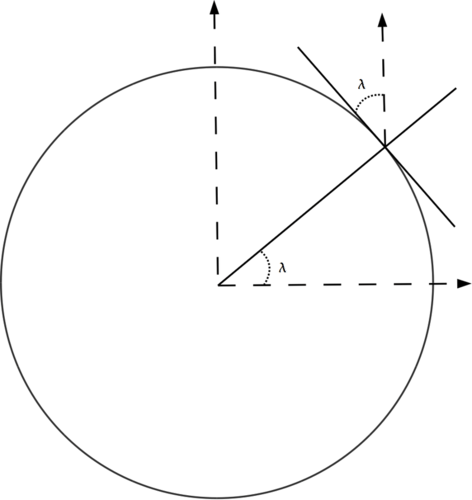11.2: Finding Latitude with the Pole Star

•• Contributed by CK12
• CK12

Imagine yourself standing at night at point P on Earth and observing the pole star (or better, the position of the north celestial pole, near that star), at an elevation angle $$\lambda$$ above the horizon.Figure $$\PageIndex{1}$$: The angle of the pole star above the horizon equals the local latitude.

The angle between the direction of the pole and the zenith is then 90−$$\lambda$$ degrees. If you continue the line from zenith downwards it reaches the center of the Earth, and the angle between it and the Earth's axis is also 90−$$\lambda$$.

Therefore, as the accompanying figure shows, $$\lambda$$ is also your latitude.